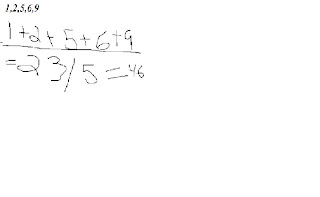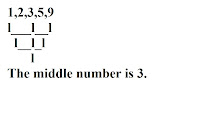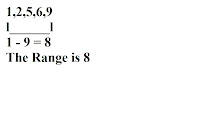### renz's measures of central tendency

Monday, September 29, 2008Mean:

• arrange the data in ascending numerical order

• add up all the data
• divide the number of data to the sum of all dataMedian

• arrange in ascending numerical order

• find the middle nuber of the dataMode

• arrange in ascending numerical order

• find the most occuring number(s) of the dataRange

• arrange in ascending numerical order

• subtract the smallest number with largest number

Here is a video about mmm.